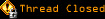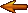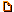# Topic awaiting preservation: JS dates.....help..? (Page 1 of 1)pwhitrow
Bipolar (III) Inmate

From: UK
Insane since: Feb 2002posted 11-22-2002 08:41

Hi all,

Small question and probably a simple one...

I have a date in a string (dateStr) of value '22/11/2002 03:00:00'.
I need to subtract 6 (or whatever) hours from that str and return the value (newStr='21/11/2002 21:00:00').
Finally, I then want to compare another date string (str1) to newDate and verify that str1 is not less than newStr.

So basically, I want to check that a date string in format dd/mm/yyyy hh24:mi:ss is not less than 6 hours old.

Can anuone help?

Regards,

pwhitrow

www.pwhitrow.com

andy_j
Nervous Wreck (II) Inmate

From: uk
Insane since: Aug 2002posted 11-26-2002 03:36

Hi Paul

A little tricky as the date format you are using is not the standard JS one.

You could do this by splitting the string and then subtracting your 6 hours and then checking the last string etc etc. It would be difficult though as you would have to work out the number of days in the month etc if the 6 hours took it back over midnight (as in your example)..

My thinking was to use j'script to do all the work for me using proper date objects. I can then use setHours() and getHours() to make the adjustments and the days, months etc will be done automatically. It would have been easier to start off with a standard format, but i am guessing you are pulling this froma db or something..

So i have come up with this script. I have used text boxes to enter and show the values but these could easily be pulled and returned to a DB or PHP script etc..

<html>
<script language="JavaScript">
function doTime() {

//The adjustable hours, ie the amount you want subtracted..

// The fixed date you want to compare others to.
var dOne = document.forms.one.value;

//First lets swap the day and month to a valid format (ie from dd,mm,yyyy hh:mm:ss to mm,dd,yyyy hh:mm:ss)
var t = dOne.split("/");
var eOne = t;
var eTwo = t;
t = eTwo;
t = eOne;
dOne = t.join("/");

//get the date/time in milliseconds
var mOne = Date.parse(dOne);

//And rebuild into a date object and copy to a second var
var fOne = new Date(mOne);
var fTwo = new Date(mOne);

//Now adjust the second date obj by the amount of hours to remove and return the amended date/time

//Now to return the amended Date/Time in the format we want, we must split fTwo down. The calc function simply adds a 0 in front of the number if its less than 10. ie the seconds 4 become 04.

function calc(n) {
n < 10 ? n = "0"+n : n;
return n
}

var u = calc(fTwo.getDate());
var v = calc(fTwo.getMonth()+1);
var w = calc(fTwo.getFullYear());
var x = calc(fTwo.getHours());
var y = calc(fTwo.getMinutes());
var z = calc(fTwo.getSeconds());
var res = u+"/"+v+"/"+w+ " "+x+":"+y+":"+z;
document.forms.result.value=res;

//Finally we will take our last string, convert to date and check to see if its older than our amened date

dTwo = document.forms.check.value;

//Again, we swap the day and month to a valid format
var b = dTwo.split("/");
var gOne = b;
var gTwo = b;
b = gTwo;
b = gOne;
dTwo = b.join("/");

//get the date/time in milliseconds
var mTwo = Date.parse(dTwo);

//Create another new date object using our final string to check
var fThr = new Date(mTwo);

//Finally check this date agaist fTwo which was our amended date/time (minus the 6 hours or whatever).
if (fThr<fTwo) {
} else {
alert("Thanks - the date/time is ok");
}
}

</script>

<body>

<form action="javascript: doTime()">
Inital Date/Time: <input type="text" name="one" value="23/11/2002 23:45:00"><br />
Hours to Remove: <input type="text" value="6" name="val"><br />
Resultant Date/Time: <input type="text" name="result"><br /><br />
Date/Time to Check: <input type="text" name="check" value="23/11/2002 12:45:00">
<input type="submit" value="run">
<br />
</form>

</body>
</html>

The first text box is the initial date/time. The second is the hours to remove and the third is the resultant.

The fourth is your time to check.

[This message has been edited by andy_j (edited 11-26-2002).]

Slime

From: Massachusetts, USA
Insane since: Mar 2000posted 11-26-2002 03:45

To extract the date from that string you have there, you might try a regular expression.

Then use the Date object.

pwhitrow
Bipolar (III) Inmate

From: UK
Insane since: Feb 2002posted 11-26-2002 15:16

Thanks Guys,

I managed to sort it. Probably not the best script in the world, but works quite well...

<script language="JavaScript">
<!--

// Compare dates of format "04/05/2002 07:00:00"

var numHours = 6

function toUKdate(what){
d = what.getDate()
m = what.getMonth()+1;
y = what.getYear()
if(d<10){d="0"+d}else{d=""+d}
if(m<10){m="0"+m}else{m=""+m}
if(y<2000){y="19"+y}else{y=""+y}
var timeStr=''
if (what.getHours() < 10){timeStr+="0"+what.getHours()+":"}else{timeStr+=what.getHours()+":"}
if (what.getMinutes() < 10){timeStr+="0"+what.getMinutes()+":"}else{timeStr+=what.getMinutes()+":"}
if (what.getSeconds() < 10){timeStr+="0"+what.getSeconds()}else{timeStr+=what.getSeconds()}
return d+'/'+m+'/'+y+' '+timeStr
}

function dateObj(s) {
var d = new Date(0);
var dd = Number(s.substring(0,2));
var m = Number(s.substring(3,5));
var y = Number(s.substring(6,10));
var hh = Number(s.substring(11,13));
var mi = Number(s.substring(14,16));
var ss = Number(s.substring(17,19));
d.setFullYear(y);
d.setMonth(m);
d.setDate(dd);
d.setHours(hh);
d.setMinutes(mi);
d.setSeconds(ss);
return d;
}

function toStdDate(d){
return new Date(d.substring(3,6)+d.substring(0,2)+d.substring(5))
}

function lastMsgDateCompare(tmpDate1,tmpDate2){
return (dateObj(tmpDate1)<dateObj(toUKdate(new Date(toStdDate(tmpDate2)-numHours*60*60*1000))));
}

//-->
</script>

www.pwhitrow.com

Slimeposted 11-26-2002 17:11